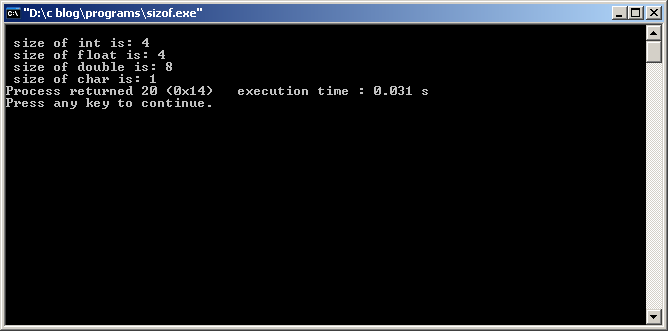# C Program example for sizeof operator

C Program To Find the Size of Data Types using sizeof operator

```#include<stdio.h>
void main()
{
printf("\n size of int is: %d",sizeof(int));
printf("\n size of float is: %d",sizeof(float));
printf("\n size of double is: %d",sizeof(double));
printf("\n size of char is: %d",sizeof(char));
}```

Program can also be written using sizeof operator with variables as given below.

```#include<stdio.h>
void main()
{
int i;
float j;
double k;
char l;
printf("\n size of int variable is: %d",sizeof(i));
printf("\n size of float variable is: %d",sizeof(j));
printf("\n size of double variable is: %d",sizeof(k));
printf("\n size of char variable is: %d",sizeof(l));
}```

Output: ( using GNU GCC Compiler with Code Blocks IDE )
Note: In borland C or Turbo C compilers size of int is 2.